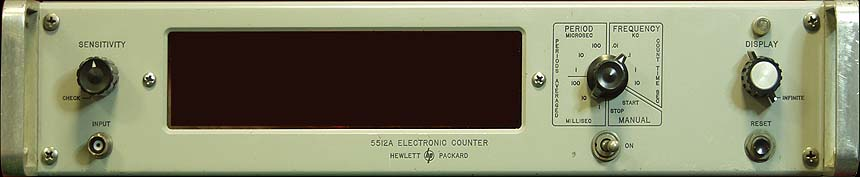## HP Clock## Wednesday, February 15, 2012

### How Many Bits Can You Use?

I have often wondered at what point the digital conversion process for audio reaches a point of demensishing returns, or at least unrealized expectations in the performance of the converters.  We live in a noisey world, and even before digital audio noise in electronics has always been with us and has always been the enemy of dynamic range.  Noises generated internally in electronic circuits are produced mostly by molecular activity. The random motion of electrons is directly related to the temperature of the conductors, and components that make up a circuit. That thermal noise generated is well understood and can be expressed in the equation as:
(eq. 1)

K = Boltzman’s constant 1.38 x 10 –23 joules per degree Kelvin
T = absolute temperature in degrees Kelvin
R = equivalent load resistance across which Et is measured
Δf = bandwidth in Hertz

Substituting 293 degrees (room temperature in degrees Kelvin), 20 Khz bandwidth, and an r of 600Ω s Et can be calculated as .22 μvolts. Voltage can be solved for where Dbm is defined as one mili-watt across 600Ω.

(eq. 2)

(eq. 3)
Inserting the .22 micro volts gives a noise floor of -130.9 db.  From here the number of bits required to digitize information can be calculated.
(eq. 4)
This works out to 21.8 bits. The digital resolution based on the number of bits can be solved for with the formula:
(eq. 5)

If we could create a converter that defied the laws of physics, and in particular the laws of thermo dynamics, one could expect a 24-bit converter to have a dynamic range of 144 db. Remember, that at 96 kHz sampling the delta f is doubled increasing the noise floor to .311 μvolts or a dynamic range of 127.9 db, which would require 21.3 bits. For a 192 kHz sample rate the bandwidth is about 80 kHz, raising the noise floor to .44 μvolts. Dynamic range is 124.9 db, with 20.8 bits as the theoretical limit.

It’s also important to note that these are calculations based on an ideal noise free environment and do not include any provision for shot noise. Like thermal noise, shot noise is a function of the electron charge, the magnitude of the current, and the bandwidth of the circuit under test. This noise occurs at boundaries where the conducted electron must cross from one type of material to another. This would include things like Vacuum tubes and solid-state junctions. Shot noise is usually expressed as a current. Even here it can be seen that the addition of bandwidth will increase the noise and reduce the dynamic range.

(eq. 6)
e = The charge of one electron (1.6 x 10 to –19th)
I = The current through the junction in amperes
Δf = bandwidth in Hertz

Realistic Analog Playback

The average analog deck outputs +4 dbm at zero VU. This translates into a voltage of 1.23 volts. Lets assume that there will be peak excursions 16 db above the zero vu setting for a total of 20 db above zero dbm. This would mean a maximum voltage of about 7.75 volts. Now lets imagine an analog tape deck working perfectly with Dolby SR noise reduction such that the signal out has a dynamic range of 85 db. Subtract the 20 db from this figure to arrive at a noise floor of -65 dbm. This would mean the smallest signal one would expect to see would be on the order of 436 μvolts If we accept the 24-bit converter can digitize to a theoretical maximum accuracy of between 20 and 22 bits, then the resolution will be between 7.39 μvolts and 1.85 μvolts.

(eq. 7)
Using the mean of 21 bits for individual transitions of 3.7 μvolts the lowest expected signal could be digitized to within 117.8 discreet values.

(eq. 8)
The number of bits can be calculated from the number of symbols assuming all symbols are equi-probable from the standard information theory equation for entropy in bits per symbol.

(eq. 9)

This would give a value of 6.88 bits per symbol at the lowest analog signal level. As a point of comparison the same 436 μvolts signal would be represented by only 3.68 symbols or 1.88 bits with 16 bit digitization.  So where does this leave us?  As you can see anything past 21 bits is past the physical limits of what is achievable by any digital conversion process.  Although some may feel that human perception extends beyond 40 KHz they cannot dispute that added bandwidth can reduce the ability of a digital converter to operate at it's maximum bit depth.  The loss of one bit in bit depth translates into one half of the resolution of that digitizing process.